ROOT   Reference Guide
Searching...
No Matches
TRatioPlot Class Reference

Class for displaying ratios, differences and fit residuals.

TRatioPlot has two constructors, one which accepts two histograms, and is responsible for setting up the calculation of ratios and differences. This calculation is in part delegated to TEfficiency. A single option can be given as a parameter, that is used to determine which procedure is chosen. The remaining option string is then passed through to the calculation, if applicable. The other constructor uses a fitted histogram to calculate the fit residual and plot it with the histogram and the fit function.

## Ratios and differences

The simplest case is passing two histograms without specifying any options. This defaults to using TGraphAsymmErrors::Divide. The option variable is passed through, as are the parameters c1 and c2, that you can set via TRatioPlot::SetC1 and TRatioPlot::SetC1. If you set the option to divsym the method TH1::Divide will be used instead, also receiving all the parameters.

Using the option diff or diffsig, both histograms will be subtracted, and in the case of diffsig, the difference will be divided by the uncertainty. c1 and c2 will only be used to scale the histograms using TH1::Scale prior to subtraction.

Available options are for option:

Option Description
divsym uses the histogram TH1::Divide method, yields symmetric errors
diff subtracts the histograms
diffsig subtracts the histograms and divides by the uncertainty
void ratioplot1() {
auto C = new TCanvas("C", "A ratio example");
auto h1 = new TH1D("h1", "TRatioPlot Example; x; y", 50, 0, 10);
auto h2 = new TH1D("h2", "h2", 50, 0, 10);
auto f1 = new TF1("f1", "exp(- x/ )");
f1->SetParameter(0, 3);
h1->FillRandom("f1", 1900);
h2->FillRandom("f1", 2000);
h1->Sumw2();
h2->Scale(1.9 / 2.);
h2->SetLineColor(kRed);
// Create and draw the ratio plot
auto rp = new TRatioPlot(h1, h2);
C->SetTicks(0, 1);
rp->Draw();
rp->GetLowYaxis()->SetNdivisions(505);
// Add a legend to the ratio plot
TLegend *legend = new TLegend(0.3,0.7,0.7,0.85);
legend->Draw();
}
@ kRed
Definition Rtypes.h:66
R__EXTERN TStyle * gStyle
Definition TStyle.h:433
The Canvas class.
Definition TCanvas.h:23
1-Dim function class
Definition TF1.h:213
virtual void SetParameter(Int_t param, Double_t value)
Definition TF1.h:639
1-D histogram with a double per channel (see TH1 documentation)}
Definition TH1.h:620
virtual void FillRandom(const char *fname, Int_t ntimes=5000, TRandom *rng=nullptr)
Fill histogram following distribution in function fname.
Definition TH1.cxx:3516
virtual void Sumw2(Bool_t flag=kTRUE)
Create structure to store sum of squares of weights.
Definition TH1.cxx:8889
This class displays a legend box (TPaveText) containing several legend entries.
Definition TLegend.h:23
TLegendEntry * AddEntry(const TObject *obj, const char *label="", Option_t *option="lpf")
Add a new entry to this legend.
Definition TLegend.cxx:317
void Draw(Option_t *option="") override
Draw this legend with its current attributes.
Definition TLegend.cxx:422
TRatioPlot()
TRatioPlot default constructor.
void SetOptStat(Int_t stat=1)
The type of information printed in the histogram statistics box can be selected via the parameter mod...
Definition TStyle.cxx:1636
TH1F * h1
Definition legend1.C:5
TF1 * f1
Definition legend1.C:11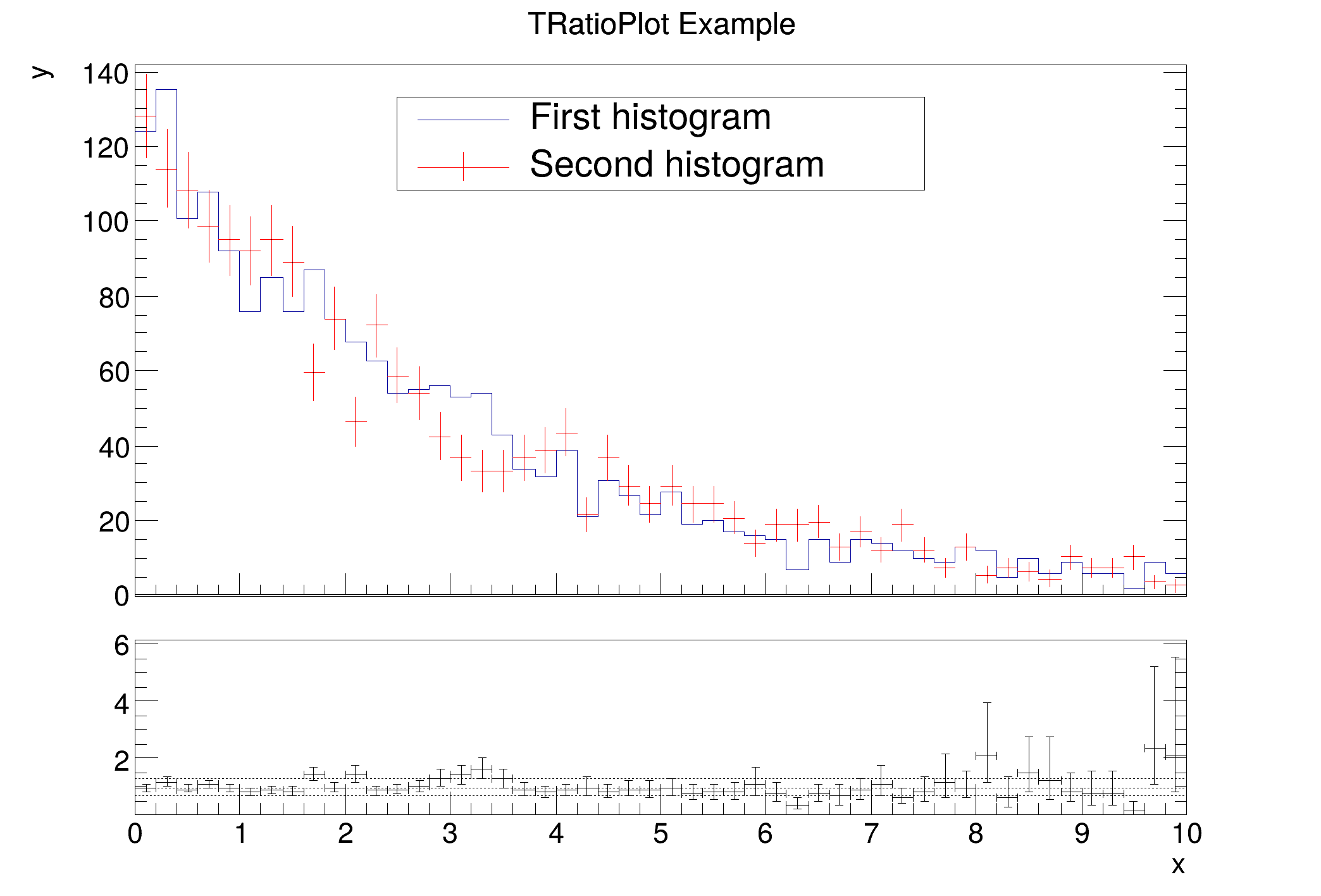## Fit residuals

A second constructor only accepts a single histogram, but expects it to have a fitted function. The function is used to calculate the residual between the fit and the histogram. Here, it is expected that h1 has a fit function in it's list of functions. The class calculates the difference between the histogram and the fit function at each point and divides it by the uncertainty. There are a few option to steer which error is used (as is the case for diffsig). The default is to use the statistical uncertainty from h1 using TH1::GetBinError. If the option string contains errasym, asymmetric errors will be used. The type of error can be steered by TH1::SetBinErrorOption. The corresponding error will be used, depending on if the function is below or above the bin content. The third option errfunc uses the square root of the function value as the error.

void ratioplot2() {
auto c1 = new TCanvas("c1", "fit residual simple");
auto h1 = new TH1D("h1", "h1", 50, -5, 5);
h1->FillRandom("gaus", 2000);
h1->Fit("gaus", "0");
h1->GetXaxis()->SetTitle("x");
auto rp1 = new TRatioPlot(h1);
rp1->Draw();
rp1->GetLowerRefYaxis()->SetTitle("ratio");
rp1->GetUpperRefYaxis()->SetTitle("entries");
}
TAxis * GetXaxis()
Definition TH1.h:322
virtual TFitResultPtr Fit(const char *formula, Option_t *option="", Option_t *goption="", Double_t xmin=0, Double_t xmax=0)
Fit histogram with function fname.
Definition TH1.cxx:3897
virtual void SetTitle(const char *title="")
Set the title of the TNamed.
Definition TNamed.cxx:164
return c1
Definition legend1.C:41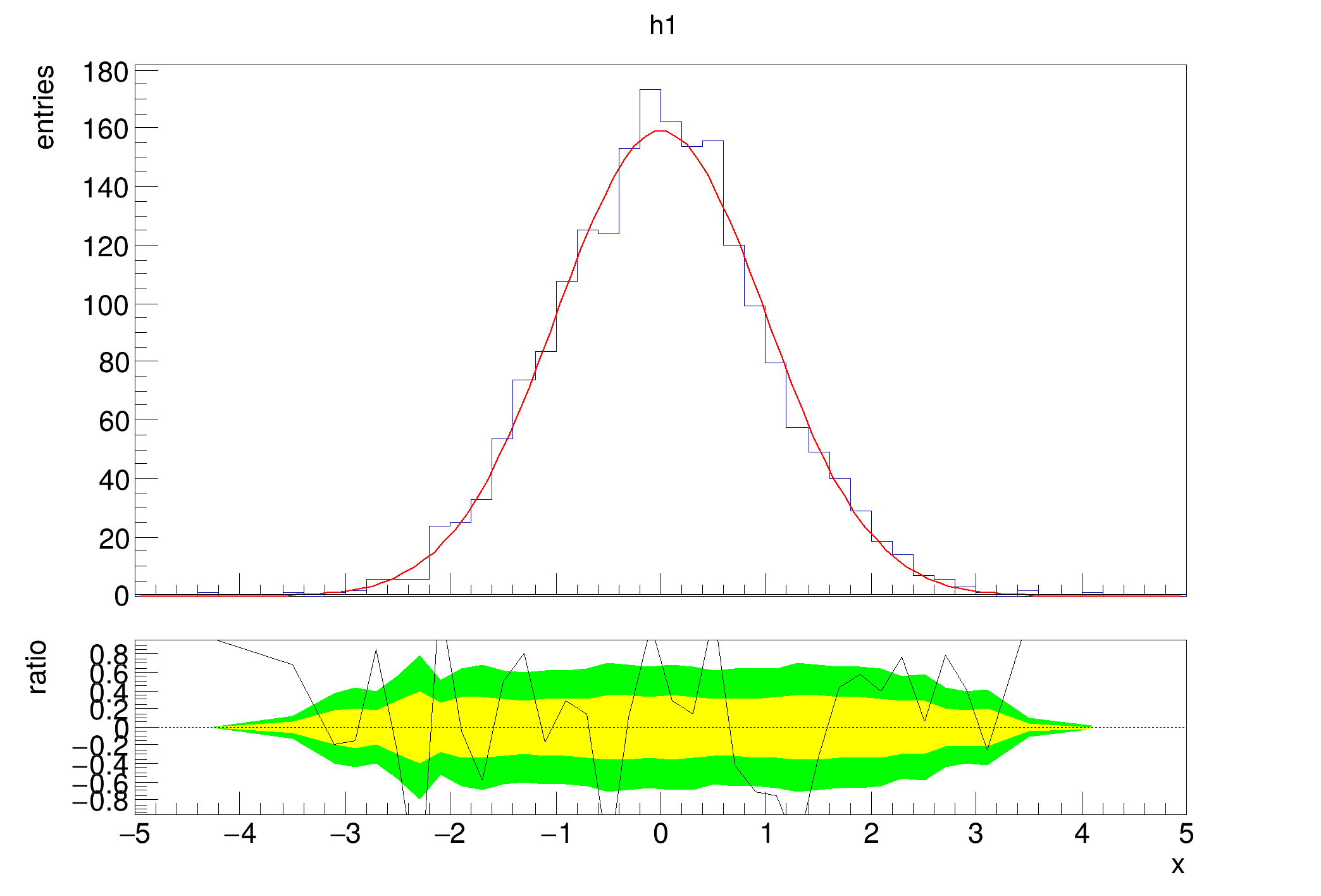## Error options for difference divided by uncertainty and fit residual

The uncertainty that is used in the calculation can be steered by providing options to the option argument.

Option Description
errasym Uses calculated asymmetric errors from TH1::GetBinErrorUp/TH1::GetBinErrorLow. Note that you need to set TH1::SetBinErrorOption first
errfunc Uses $$\sqrt{f(x)}$$ as the error

The asymmetric error case uses the upper or lower error depending on the relative size of the bin contents, or the bin content and the function value.

You can access the internal objects that are used to construct the plot via a series of methods. TRatioPlot::GetUpperPad and TRatioPlot::GetLowerPad can be used to draw additional elements on top of the existing ones. TRatioPlot::GetLowerRefGraph returns a reference to the lower pad's graph that is responsible for the range, which enables you to modify the range.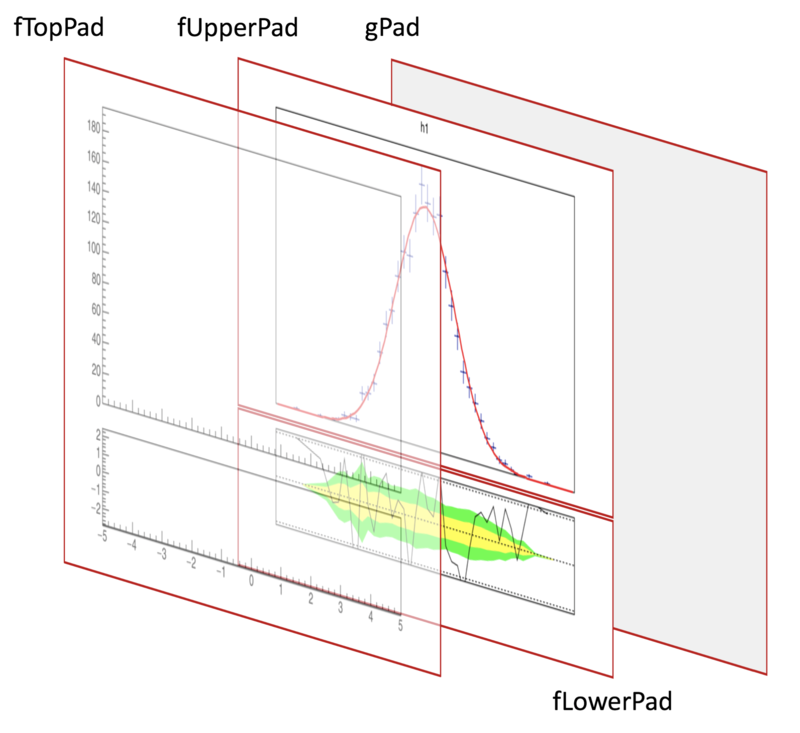Definition at line 43 of file TRatioPlot.h.

## Public Member Functions

TRatioPlot ()
TRatioPlot default constructor.

TRatioPlot (TH1 *h1, Option_t *option="", TFitResult *fitres=nullptr)
Constructor for one histogram and a fit.

TRatioPlot (TH1 *h1, TH1 *h2, Option_t *option="pois")
Constructor for two histograms.

TRatioPlot (THStack *st, TH1 *h2, Option_t *option="pois")
Constructor which accepts a THStack and a histogram.

~TRatioPlot () override
Destructor.

void Browse (TBrowser *b) override
Browse.

void Draw (Option_t *chopt="") override
Draws the ratio plot to the currently active pad.

TGraphGetCalculationOutputGraph () const
Get the output of the calculation in the form of a graph.

TGraphErrorsGetConfidenceInterval1 () const
Returns the graph for the 1 sigma confidence interval in the fit residual case.

TGraphErrorsGetConfidenceInterval2 () const
Returns the graph for the 2 sigma confidence interval in the fit residual case.

virtual TGraphGetLowerRefGraph () const
Returns the reference graph for the lower pad, which means the graph that is responsible for setting the coordinate system.

TAxisGetLowerRefXaxis () const
Shortcut for:

TAxisGetLowerRefYaxis () const
Shortcut for:

TAxisGetLowYaxis () const

Float_t GetSeparationMargin () const
Return the separation margin value.

virtual TObjectGetUpperRefObject () const
Return the reference object.

TAxisGetUpperRefXaxis () const
Gets the x axis of the object returned by TRatioPlot::GetUpperRefObject.

TAxisGetUpperRefYaxis () const
Gets the y axis of the object returned by TRatioPlot::GetUpperRefObject.

TAxisGetUpYaxis () const

TAxisGetXaxis () const

TClassIsA () const override

void Paint (Option_t *opt="") override
Creates the visual axes when painting.

void RangeAxisChanged ()
Slot that receives the RangeAxisChanged signal from any of the pads and reacts correspondingly.

void SetC1 (Double_t c1)

void SetC2 (Double_t c2)

void SetConfidenceIntervalColors (Color_t ci1=kYellow, Color_t ci2=kGreen)
Set the confidence interval colors.

void SetConfidenceLevels (Double_t cl1, Double_t cl2)
Sets the confidence levels used to calculate the bands in the fit residual case.

void SetFitDrawOpt (Option_t *opt)
Sets the drawing option for the fit in the fit residual case.

void SetFitResult (TFitResultPtr fitres)
Explicitly specify the fit result that is to be used for fit residual calculation.

void SetGraphDrawOpt (Option_t *opt)
Sets the drawing option for the lower graph.

virtual void SetGridlines (Double_t *gridlines, Int_t numGridlines)
Set where horizontal, dashed lines are drawn on the lower pad.

virtual void SetGridlines (std::vector< double > gridlines)
Set where horizontal, dashed lines are drawn on the lower pad.

void SetH1DrawOpt (Option_t *opt)
Sets the drawing option for h1.

void SetH2DrawOpt (Option_t *opt)
Sets the drawing option for h2.

void SetInsetWidth (Double_t width)
Set the inset on the outer sides of all the pads.

void SetLeftMargin (Float_t margin)
Sets the left margin of both pads.

void SetLowBottomMargin (Float_t margin)
Sets the bottom margin of the lower pad.

void SetLowTopMargin (Float_t margin)
Sets the top margin of the lower pad.

void SetRightMargin (Float_t margin)
Sets the right margin of both pads.

void SetSeparationMargin (Float_t)
Sets the margin that separates the two pads.

void SetSplitFraction (Float_t sf)
Set the fraction of the parent pad, at which the to sub pads should meet.

void SetUpBottomMargin (Float_t margin)
Sets the bottom margin of the upper pad.

void SetUpTopMargin (Float_t margin)
Sets the top margin of the upper pad.

void Streamer (TBuffer &) override
Stream an object of class TObject.

void StreamerNVirtual (TBuffer &ClassDef_StreamerNVirtual_b)

Slot that handles common resizing of upper and lower pad.

void UnZoomed ()
Slot for the UnZoomed signal that was introduced to TAxis.Public Member Functions inherited from TObject
TObject ()
TObject constructor.

TObject (const TObject &object)
TObject copy ctor.

virtual ~TObject ()
TObject destructor.

void AbstractMethod (const char *method) const
Use this method to implement an "abstract" method that you don't want to leave purely abstract.

Append graphics object to current pad.

ULong_t CheckedHash ()
Check and record whether this class has a consistent Hash/RecursiveRemove setup (*) and then return the regular Hash value for this object.

virtual const char * ClassName () const
Returns name of class to which the object belongs.

virtual void Clear (Option_t *="")

virtual TObjectClone (const char *newname="") const
Make a clone of an object using the Streamer facility.

virtual Int_t Compare (const TObject *obj) const
Compare abstract method.

virtual void Copy (TObject &object) const
Copy this to obj.

virtual void Delete (Option_t *option="")
Delete this object.

virtual Int_t DistancetoPrimitive (Int_t px, Int_t py)
Computes distance from point (px,py) to the object.

virtual void DrawClass () const
Draw class inheritance tree of the class to which this object belongs.

virtual TObjectDrawClone (Option_t *option="") const
Draw a clone of this object in the current selected pad with: gROOT->SetSelectedPad(c1).

virtual void Dump () const
Dump contents of object on stdout.

virtual void Error (const char *method, const char *msgfmt,...) const
Issue error message.

virtual void Execute (const char *method, const char *params, Int_t *error=nullptr)
Execute method on this object with the given parameter string, e.g.

virtual void Execute (TMethod *method, TObjArray *params, Int_t *error=nullptr)
Execute method on this object with parameters stored in the TObjArray.

virtual void ExecuteEvent (Int_t event, Int_t px, Int_t py)
Execute action corresponding to an event at (px,py).

virtual void Fatal (const char *method, const char *msgfmt,...) const
Issue fatal error message.

virtual TObjectFindObject (const char *name) const
Must be redefined in derived classes.

virtual TObjectFindObject (const TObject *obj) const
Must be redefined in derived classes.

virtual Option_tGetDrawOption () const
Get option used by the graphics system to draw this object.

virtual const char * GetIconName () const
Returns mime type name of object.

virtual const char * GetName () const
Returns name of object.

virtual char * GetObjectInfo (Int_t px, Int_t py) const
Returns string containing info about the object at position (px,py).

virtual Option_tGetOption () const

virtual const char * GetTitle () const
Returns title of object.

virtual UInt_t GetUniqueID () const
Return the unique object id.

virtual Bool_t HandleTimer (TTimer *timer)
Execute action in response of a timer timing out.

virtual ULong_t Hash () const
Return hash value for this object.

Bool_t HasInconsistentHash () const
Return true is the type of this object is known to have an inconsistent setup for Hash and RecursiveRemove (i.e.

virtual void Info (const char *method, const char *msgfmt,...) const
Issue info message.

virtual Bool_t InheritsFrom (const char *classname) const
Returns kTRUE if object inherits from class "classname".

virtual Bool_t InheritsFrom (const TClass *cl) const
Returns kTRUE if object inherits from TClass cl.

virtual void Inspect () const
Dump contents of this object in a graphics canvas.

void InvertBit (UInt_t f)

Bool_t IsDestructed () const
IsDestructed.

virtual Bool_t IsEqual (const TObject *obj) const
Default equal comparison (objects are equal if they have the same address in memory).

virtual Bool_t IsFolder () const
Returns kTRUE in case object contains browsable objects (like containers or lists of other objects).

R__ALWAYS_INLINE Bool_t IsOnHeap () const

virtual Bool_t IsSortable () const

R__ALWAYS_INLINE Bool_t IsZombie () const

virtual void ls (Option_t *option="") const
The ls function lists the contents of a class on stdout.

void MayNotUse (const char *method) const
Use this method to signal that a method (defined in a base class) may not be called in a derived class (in principle against good design since a child class should not provide less functionality than its parent, however, sometimes it is necessary).

virtual Bool_t Notify ()
This method must be overridden to handle object notification (the base implementation is no-op).

void Obsolete (const char *method, const char *asOfVers, const char *removedFromVers) const
Use this method to declare a method obsolete.

void operator delete (void *ptr)
Operator delete.

void operator delete[] (void *ptr)
Operator delete [].

void * operator new (size_t sz)

void * operator new (size_t sz, void *vp)

void * operator new[] (size_t sz)

void * operator new[] (size_t sz, void *vp)

TObjectoperator= (const TObject &rhs)
TObject assignment operator.

virtual void Pop ()
Pop on object drawn in a pad to the top of the display list.

virtual void Print (Option_t *option="") const
This method must be overridden when a class wants to print itself.

virtual Int_t Read (const char *name)
Read contents of object with specified name from the current directory.

virtual void RecursiveRemove (TObject *obj)
Recursively remove this object from a list.

void ResetBit (UInt_t f)

virtual void SaveAs (const char *filename="", Option_t *option="") const
Save this object in the file specified by filename.

virtual void SavePrimitive (std::ostream &out, Option_t *option="")
Save a primitive as a C++ statement(s) on output stream "out".

void SetBit (UInt_t f)

void SetBit (UInt_t f, Bool_t set)
Set or unset the user status bits as specified in f.

virtual void SetDrawOption (Option_t *option="")
Set drawing option for object.

virtual void SetUniqueID (UInt_t uid)
Set the unique object id.

void StreamerNVirtual (TBuffer &ClassDef_StreamerNVirtual_b)

virtual void SysError (const char *method, const char *msgfmt,...) const
Issue system error message.

R__ALWAYS_INLINE Bool_t TestBit (UInt_t f) const

Int_t TestBits (UInt_t f) const

virtual void UseCurrentStyle ()
Set current style settings in this object This function is called when either TCanvas::UseCurrentStyle or TROOT::ForceStyle have been invoked.

virtual void Warning (const char *method, const char *msgfmt,...) const
Issue warning message.

virtual Int_t Write (const char *name=nullptr, Int_t option=0, Int_t bufsize=0)
Write this object to the current directory.

virtual Int_t Write (const char *name=nullptr, Int_t option=0, Int_t bufsize=0) const
Write this object to the current directory.

## Static Public Member Functions

static TClassClass ()

static const char * Class_Name ()

static constexpr Version_t Class_Version ()

static const char * DeclFileName ()Static Public Member Functions inherited from TObject
static TClassClass ()

static const char * Class_Name ()

static constexpr Version_t Class_Version ()

static const char * DeclFileName ()

static Longptr_t GetDtorOnly ()
Return destructor only flag.

static Bool_t GetObjectStat ()
Get status of object stat flag.

static void SetDtorOnly (void *obj)
Set destructor only flag.

static void SetObjectStat (Bool_t stat)
Turn on/off tracking of objects in the TObjectTable.

## Protected Member Functions

Int_t BuildLowerPlot ()
Build the lower plot according to which constructor was called, and which options were passed.

void CreateGridline ()
Create a grid line.

virtual void CreateVisualAxes ()
(Re-)Creates the TGAxis objects that are used for consistent display of the axes.

void ImportAxisAttributes (TGaxis *gaxis, TAxis *axis)
Internal method to import TAxis attributes to a TGaxis.

virtual void Init (TH1 *h1, TH1 *h2, Option_t *option="")
Internal method that shares constructor logic.

Bool_t IsDrawn ()
Check if ... is drawn.

Sets the margins of all the pads to the value specified in class members.

virtual void SyncAxesRanges ()
Syncs the axes ranges from the shared ones to the actual ones.

Figures out which pad margin has deviated from the stored ones, to figure out what the new nominal is and set the other pad to it subsequently.Protected Member Functions inherited from TObject
virtual void DoError (int level, const char *location, const char *fmt, va_list va) const
Interface to ErrorHandler (protected).

void MakeZombie ()

## Protected Attributes

Double_t fC1 = 1.
Stores the scale factor for h1 (or THStack sum)

Double_t fC2 = 1.
Stores the scale factor for h2.

Color_t fCi1Color = kYellow
Stores the color for the 1 sigma band.

Color_t fCi2Color = kGreen
Stores the color for the 2 sigma band.

Double_t fCl1 = 0.6827
Stores the confidence level for the inner confidence interval band.

Double_t fCl2 = 0.9545
Stores the confidence level for the outer confidence interval band.

TGraphErrorsfConfidenceInterval1 = nullptr
Stores the graph for the 1 sigma band.

TGraphErrorsfConfidenceInterval2 = nullptr
Stores the graph for the 2 sigma band.

Int_t fErrorMode = TRatioPlot::ErrorMode::kErrorSymmetric
Stores the error mode, sym, asym or func.

TString fFitDrawOpt = ""
Stores draw option for the fit function in the fit residual case.

TFitResultfFitResult = nullptr
Stores the explicit fit result given in the fit residual case. Can be 0.

TString fGraphDrawOpt = ""
Stores draw option for the lower plot graph given in constructor.

std::vector< doublefGridlinePositions
Stores the y positions for the gridlines.

std::vector< TLine * > fGridlines
Keeps TLine objects for the gridlines.

TH1fH1 = nullptr
Stores the primary histogram.

TString fH1DrawOpt = ""
Stores draw option for h1 given in constructor.

TH1fH2 = nullptr
Stores the secondary histogram, if there is one.

TString fH2DrawOpt = ""
Stores draw option for h2 given in constructor.

Int_t fHideLabelMode = TRatioPlot::HideLabelMode::kHideLow
Stores which label to hide if the margin is to narrow, if at all.

TObjectfHistDrawProxy = nullptr
The object which is actually drawn, this might be TH1 or THStack.

Float_t fInsetWidth = 0.0025

Keeps track whether pads are updating during resizing.

Bool_t fIsUpdating = kFALSE
Keeps track of whether its currently updating to reject other calls until done.

Float_t fLeftMargin = 0.1
Stores the common left margin of both pads.

Float_t fLowBottomMargin = 0.3
Stores the bottom margin of the lower pad.

TGaxisfLowerGXaxis = nullptr
Lower graphical x axis.

TGaxisfLowerGXaxisMirror = nullptr
Lower mirror of the x axis.

TGaxisfLowerGYaxis = nullptr
Lower graphical y axis.

TGaxisfLowerGYaxisMirror = nullptr
Lower mirror of the y axis.

The pad which contains the calculated lower plot part.

Float_t fLowTopMargin = 0.05
Stores the top margin of the lower pad.

TAxisfLowYaxis = nullptr
Clone of the lower y axis.

Int_t fMode = 0
Stores which calculation is supposed to be performed as specified by user option.

TString fOption = ""
Stores the option which is given in the constructor as a string.

Stores the pad the ratio plot was created in.

TGraphfRatioGraph = nullptr
Stores the lower plot's graph.

Float_t fRightMargin = 0.1
Stores the common right margin of both pads.

TAxisfSharedXAxis = nullptr
X axis that stores the range for both plots.

Bool_t fShowConfidenceIntervals = kTRUE
Stores whether to show the confidence interval bands. From Draw option.

Bool_t fShowGridlines = kTRUE
Stores whether to show the gridlines at all.

Float_t fSplitFraction = 0.3
Stores the fraction at which the upper and lower pads meet.

The Pad that drawn on top on the others to have consistent coordinates.

Float_t fUpBottomMargin = 0.05
Stores the bottom margin of the upper pad.

TGaxisfUpperGXaxis = nullptr
Upper graphical x axis.

TGaxisfUpperGXaxisMirror = nullptr
Upper mirror of the x axis.

TGaxisfUpperGYaxis = nullptr
Upper graphical y axis.

TGaxisfUpperGYaxisMirror = nullptr
Upper mirror of the y axis.

The pad which contains the upper plot part.

Float_t fUpTopMargin = 0.1
Stores the top margin of the upper pad.

TAxisfUpYaxis = nullptr
Clone of the upper y axis.

## Private Types

enum  CalculationMode {
kDivideHist = 1 , kDivideGraph = 2 , kDifference = 3 , kFitResidual = 4 ,
kDifferenceSign = 5
}

enum  ErrorMode { kErrorSymmetric = 1 , kErrorAsymmetric = 2 , kErrorFunc = 3 }

enum  HideLabelMode {
kHideUp = 1 , kHideLow = 2 , kNoHide = 3 , kForceHideUp = 4 ,
kForceHideLow = 5
}

## Private Member Functions

TRatioPlot (const TRatioPlot &)=delete

TRatioPlotoperator= (const TRatioPlot &)=deletePublic Types inherited from TObject
enum  {
kIsOnHeap = 0x01000000 , kNotDeleted = 0x02000000 , kZombie = 0x04000000 , kInconsistent = 0x08000000 ,
}

enum  { kSingleKey = (1ULL << ( 0 )) , kOverwrite = (1ULL << ( 1 )) , kWriteDelete = (1ULL << ( 2 )) }

enum  EDeprecatedStatusBits { kObjInCanvas = (1ULL << ( 3 )) }

enum  EStatusBits {
kCanDelete = (1ULL << ( 0 )) , kMustCleanup = (1ULL << ( 3 )) , kIsReferenced = (1ULL << ( 4 )) , kHasUUID = (1ULL << ( 5 )) ,
kCannotPick = (1ULL << ( 6 )) , kNoContextMenu = (1ULL << ( 8 )) , kInvalidObject = (1ULL << ( 13 ))
}Protected Types inherited from TObject
enum  { kOnlyPrepStep = (1ULL << ( 3 )) }

#include <TRatioPlot.h>

Inheritance diagram for TRatioPlot:
[legend]

## ◆ CalculationMode

 private
Enumerator
kDivideHist

Use TH1::Divide to create the ratio.

kDivideGraph

Use TGraphAsymmErrors::Divide to create the ratio.

kDifference

Calculate the difference between the histograms.

kFitResidual

Calculate the fit residual between the histogram and a fit stored within it.

kDifferenceSign

Calculate the difference divided by the error.

Definition at line 49 of file TRatioPlot.h.

## ◆ ErrorMode

 private
Enumerator
kErrorSymmetric

Use the regular TH1::GetBinError as the error.

kErrorAsymmetric

Use TH1::GetBinErrorUp and TH1::GetBinErrorLow for the error, depending on y values.

kErrorFunc

Use the square root of the function value as the error.

Definition at line 57 of file TRatioPlot.h.

## ◆ HideLabelMode

 private
Enumerator
kHideUp

Hide the first label of the upper y axis when there is low space.

kHideLow

Hide the last label of the lower y axis when there is low space.

kNoHide

Do not hide labels when there is low space.

kForceHideUp

Always hide the first label of the upper y axis.

kForceHideLow

Always hide the last label of the lower y axis.

Definition at line 63 of file TRatioPlot.h.

## ◆ TRatioPlot() [1/5]

 TRatioPlot::TRatioPlot ( const TRatioPlot & )
privatedelete

## ◆ TRatioPlot() [2/5]

 TRatioPlot::TRatioPlot ( )

TRatioPlot default constructor.

Definition at line 102 of file TRatioPlot.cxx.

## ◆ ~TRatioPlot()

 TRatioPlot::~TRatioPlot ( )
override

Destructor.

Definition at line 109 of file TRatioPlot.cxx.

## ◆ TRatioPlot() [3/5]

 TRatioPlot::TRatioPlot ( TH1 * h1, TH1 * h2, Option_t * option = "pois" )

Constructor for two histograms.

Parameters
 h1 First histogram h2 Second histogram option Steers the error calculation, as well as ratio / difference

Definition at line 202 of file TRatioPlot.cxx.

## ◆ TRatioPlot() [4/5]

 TRatioPlot::TRatioPlot ( THStack * st, TH1 * h2, Option_t * option = "pois" )

Constructor which accepts a THStack and a histogram.

Converts the stack to a regular sum of its containing histograms for processing.

Parameters
 st The THStack object h2 The other histogram option Steers the calculation of the lower plot

Definition at line 234 of file TRatioPlot.cxx.

## ◆ TRatioPlot() [5/5]

 TRatioPlot::TRatioPlot ( TH1 * h1, Option_t * option = "", TFitResult * fitres = nullptr )

Constructor for one histogram and a fit.

Parameters
 h1 The histogram option Steers the error calculation fitres Explicit fit result to be used for calculation. Uses last fit if left empty

Definition at line 268 of file TRatioPlot.cxx.

## ◆ Browse()

 void TRatioPlot::Browse ( TBrowser * b )
overridevirtual

Browse.

Reimplemented from TObject.

Definition at line 427 of file TRatioPlot.cxx.

## ◆ BuildLowerPlot()

 Int_t TRatioPlot::BuildLowerPlot ( )
protected

Build the lower plot according to which constructor was called, and which options were passed.

Definition at line 859 of file TRatioPlot.cxx.

## ◆ Class()

 static TClass * TRatioPlot::Class ( )
static
Returns
TClass describing this class

## ◆ Class_Name()

 static const char * TRatioPlot::Class_Name ( )
static
Returns
Name of this class

## ◆ Class_Version()

 static constexpr Version_t TRatioPlot::Class_Version ( )
inlinestaticconstexpr
Returns
Version of this class

Definition at line 264 of file TRatioPlot.h.

## ◆ CreateGridline()

 void TRatioPlot::CreateGridline ( )
protected

Create a grid line.

Definition at line 763 of file TRatioPlot.cxx.

## ◆ CreateVisualAxes()

 void TRatioPlot::CreateVisualAxes ( )
protectedvirtual

(Re-)Creates the TGAxis objects that are used for consistent display of the axes.

Definition at line 1102 of file TRatioPlot.cxx.

## ◆ DeclFileName()

 static const char * TRatioPlot::DeclFileName ( )
inlinestatic
Returns
Name of the file containing the class declaration

Definition at line 264 of file TRatioPlot.h.

## ◆ Draw()

 void TRatioPlot::Draw ( Option_t * option = "" )
overridevirtual

Draws the ratio plot to the currently active pad.

Therefore it requires that a TCanvas has been created first.

It takes the following options

Option Description
grid / nogrid enable (default) or disable drawing of dashed lines on lower plot
hideup hides the first label of the upper axis if there is not enough space
fhideup always hides the first label of the upper axis
hidelow (default) hides the last label of the lower axis if there is not enough space
fhidelow always hides the last label of the lower axis
nohide does not hide a label if there is not enough space
noconfint does not draw the confidence interval bands in the fit residual case
confint draws the confidence interval bands in the fit residual case (default)

Reimplemented from TObject.

Definition at line 544 of file TRatioPlot.cxx.

## ◆ GetCalculationOutputGraph()

 TGraph * TRatioPlot::GetCalculationOutputGraph ( ) const
inline

Get the output of the calculation in the form of a graph.

The type of the return value depends on the input option that was given in the constructor.

Definition at line 217 of file TRatioPlot.h.

## ◆ GetConfidenceInterval1()

 TGraphErrors * TRatioPlot::GetConfidenceInterval1 ( ) const
inline

Returns the graph for the 1 sigma confidence interval in the fit residual case.

Definition at line 222 of file TRatioPlot.h.

## ◆ GetConfidenceInterval2()

 TGraphErrors * TRatioPlot::GetConfidenceInterval2 ( ) const
inline

Returns the graph for the 2 sigma confidence interval in the fit residual case.

Definition at line 227 of file TRatioPlot.h.

inline

Definition at line 230 of file TRatioPlot.h.

## ◆ GetLowerRefGraph()

 TGraph * TRatioPlot::GetLowerRefGraph ( ) const
virtual

Returns the reference graph for the lower pad, which means the graph that is responsible for setting the coordinate system.

It is the first graph added to the primitive list of the lower pad. This reference can be used to set the minimum and maximum of the lower pad. Note that TRatioPlot::Draw needs to have been called first, since the graphs are only created then.

void ratioplot3() {
auto c1 = new TCanvas("c1", "fit residual simple");
c1->SetLogy();
auto h1 = new TH1D("h1", "h1", 50, -5, 5);
h1->FillRandom("gaus", 2000);
h1->Fit("gaus", "0");
h1->SetMinimum(0.001);
h1->GetXaxis()->SetTitle("x");
h1->GetYaxis()->SetTitle("y");
auto rp1 = new TRatioPlot(h1);
rp1->Draw();
rp1->GetLowerRefGraph()->SetMinimum(-2);
rp1->GetLowerRefGraph()->SetMaximum(2);
}
TAxis * GetYaxis()
Definition TH1.h:323
virtual void SetMinimum(Double_t minimum=-1111)
Definition TH1.h:401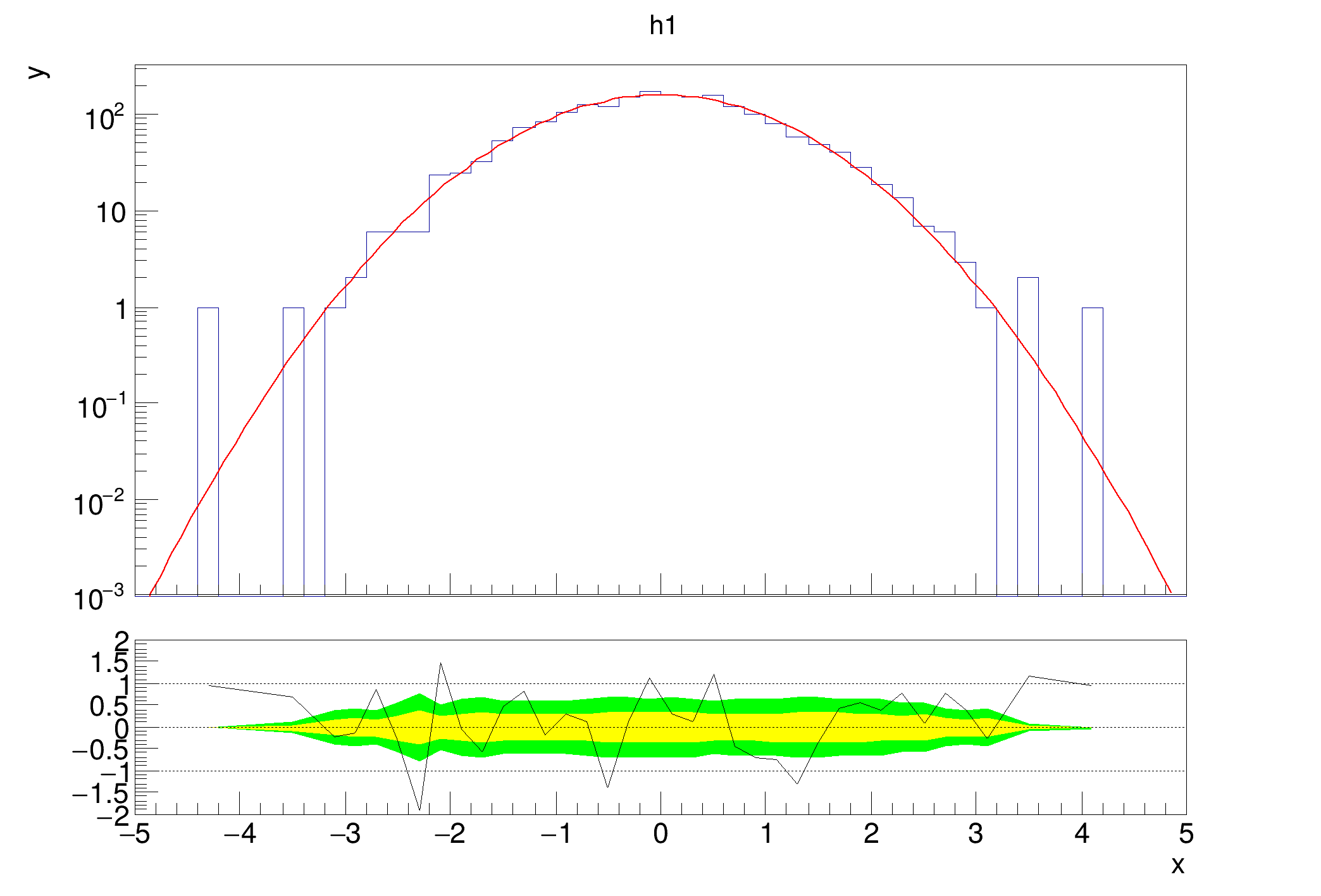Definition at line 673 of file TRatioPlot.cxx.

## ◆ GetLowerRefXaxis()

 TAxis * TRatioPlot::GetLowerRefXaxis ( ) const
inline

Shortcut for:

rp->GetLowerRefGraph()->GetXaxis();

Definition at line 198 of file TRatioPlot.h.

## ◆ GetLowerRefYaxis()

 TAxis * TRatioPlot::GetLowerRefYaxis ( ) const
inline

Shortcut for:

rp->GetLowerRefGraph()->GetYaxis();

Definition at line 207 of file TRatioPlot.h.

## ◆ GetLowYaxis()

 TAxis * TRatioPlot::GetLowYaxis ( ) const
inline

Definition at line 187 of file TRatioPlot.h.

## ◆ GetSeparationMargin()

 Float_t TRatioPlot::GetSeparationMargin ( ) const

Return the separation margin value.

Definition at line 519 of file TRatioPlot.cxx.

inline

Definition at line 229 of file TRatioPlot.h.

## ◆ GetUpperRefObject()

 TObject * TRatioPlot::GetUpperRefObject ( ) const
virtual

Return the reference object.

Its the first TH1 or THStack type object in the upper pads list of primitives. Note that it returns a TObject, so you need to test and cast it to use it.

Definition at line 707 of file TRatioPlot.cxx.

## ◆ GetUpperRefXaxis()

 TAxis * TRatioPlot::GetUpperRefXaxis ( ) const

Gets the x axis of the object returned by TRatioPlot::GetUpperRefObject.

Definition at line 725 of file TRatioPlot.cxx.

## ◆ GetUpperRefYaxis()

 TAxis * TRatioPlot::GetUpperRefYaxis ( ) const

Gets the y axis of the object returned by TRatioPlot::GetUpperRefObject.

Definition at line 744 of file TRatioPlot.cxx.

## ◆ GetUpYaxis()

 TAxis * TRatioPlot::GetUpYaxis ( ) const
inline

Definition at line 186 of file TRatioPlot.h.

## ◆ GetXaxis()

 TAxis * TRatioPlot::GetXaxis ( ) const
inline

Definition at line 185 of file TRatioPlot.h.

## ◆ ImportAxisAttributes()

 void TRatioPlot::ImportAxisAttributes ( TGaxis * gaxis, TAxis * axis )
protected

Internal method to import TAxis attributes to a TGaxis.

Copied from TGaxis::ImportAxisAttributes

Definition at line 1709 of file TRatioPlot.cxx.

## ◆ Init()

 void TRatioPlot::Init ( TH1 * h1, TH1 * h2, Option_t * option = "" )
protectedvirtual

Internal method that shares constructor logic.

Definition at line 144 of file TRatioPlot.cxx.

## ◆ IsA()

 TClass * TRatioPlot::IsA ( ) const
inlineoverridevirtual
Returns
TClass describing current object

Reimplemented from TObject.

Definition at line 264 of file TRatioPlot.h.

## ◆ IsDrawn()

 Bool_t TRatioPlot::IsDrawn ( )
protected

Check if ... is drawn.

Definition at line 1600 of file TRatioPlot.cxx.

## ◆ operator=()

 TRatioPlot & TRatioPlot::operator= ( const TRatioPlot & )
privatedelete

## ◆ Paint()

 void TRatioPlot::Paint ( Option_t * opt = "" )
overridevirtual

Creates the visual axes when painting.

Reimplemented from TObject.

Definition at line 827 of file TRatioPlot.cxx.

## ◆ RangeAxisChanged()

 void TRatioPlot::RangeAxisChanged ( )

Slot that receives the RangeAxisChanged signal from any of the pads and reacts correspondingly.

Definition at line 1460 of file TRatioPlot.cxx.

## ◆ SetC1()

 void TRatioPlot::SetC1 ( Double_t c1 )
inline

Definition at line 261 of file TRatioPlot.h.

## ◆ SetC2()

 void TRatioPlot::SetC2 ( Double_t c2 )
inline

Definition at line 262 of file TRatioPlot.h.

## ◆ SetConfidenceIntervalColors()

 void TRatioPlot::SetConfidenceIntervalColors ( Color_t ci1 = kYellow, Color_t ci2 = kGreen )

Set the confidence interval colors.

Parameters
 ci1 Color of the 1 sigma band ci2 Color of the 2 sigma band Sets the color of the 1 and 2 sigma bands in the fit residual case.
void ratioplot5() {
auto c1 = new TCanvas("c1", "fit residual simple");
auto h1 = new TH1D("h1", "h1", 50, -5, 5);
h1->FillRandom("gaus", 2000);
h1->Fit("gaus","0");
h1->GetXaxis()->SetTitle("x");
h1->GetYaxis()->SetTitle("y");
auto rp1 = new TRatioPlot(h1);
rp1->SetConfidenceIntervalColors(kBlue, kRed);
rp1->Draw();
}
@ kBlue
Definition Rtypes.h:66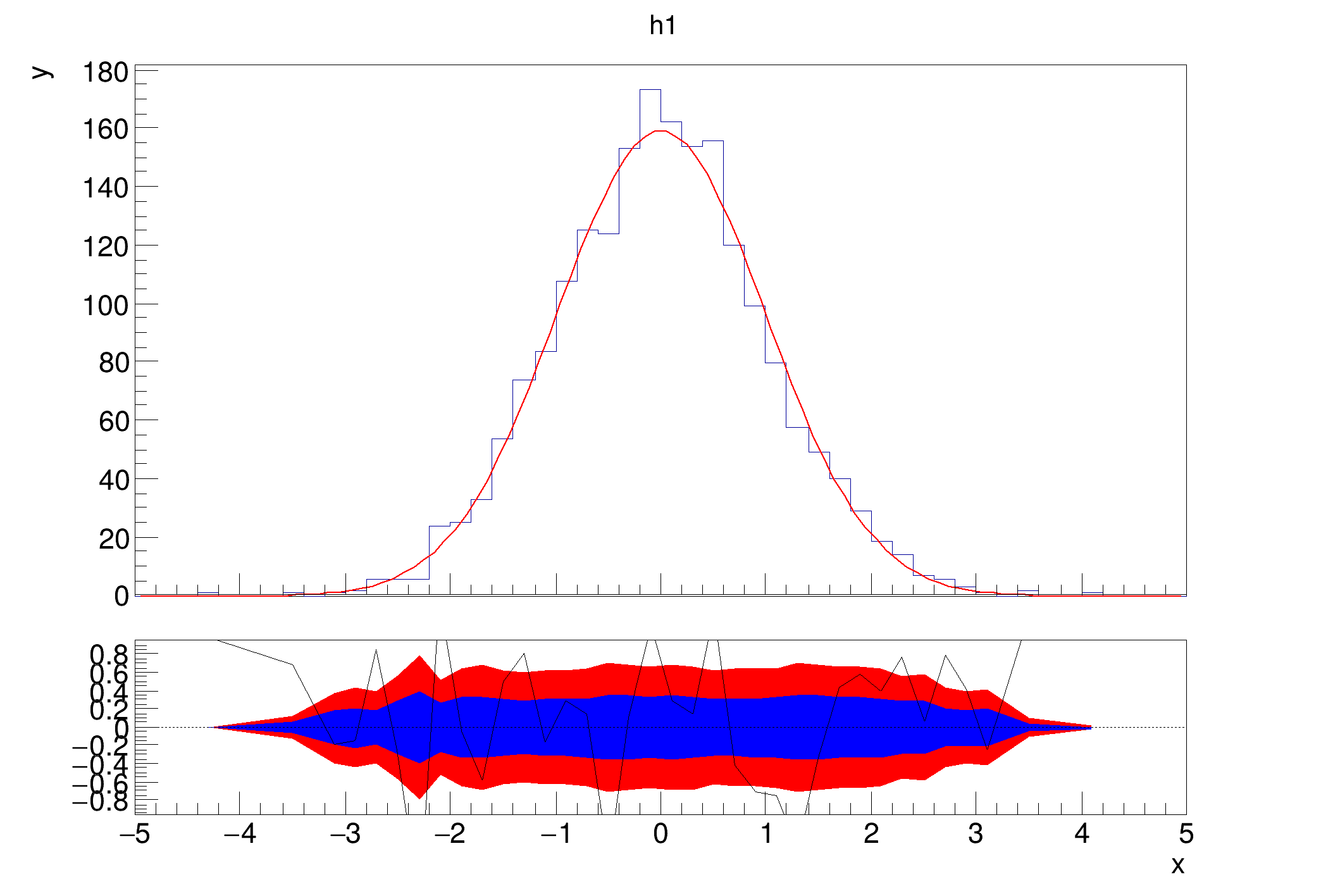Definition at line 1699 of file TRatioPlot.cxx.

## ◆ SetConfidenceLevels()

 void TRatioPlot::SetConfidenceLevels ( Double_t c1, Double_t c2 )

Sets the confidence levels used to calculate the bands in the fit residual case.

Defaults to 1 and 2 sigma.

Definition at line 1651 of file TRatioPlot.cxx.

## ◆ SetFitDrawOpt()

 void TRatioPlot::SetFitDrawOpt ( Option_t * opt )

Sets the drawing option for the fit in the fit residual case.

Definition at line 366 of file TRatioPlot.cxx.

## ◆ SetFitResult()

 void TRatioPlot::SetFitResult ( TFitResultPtr fitres )
inline

Explicitly specify the fit result that is to be used for fit residual calculation.

If it is not provided, the last fit registered in the global fitter is used. The fit result can also be specified in the constructor.

Parameters
 fitres The fit result coming from the fit function call

Definition at line 241 of file TRatioPlot.h.

## ◆ SetGraphDrawOpt()

 void TRatioPlot::SetGraphDrawOpt ( Option_t * opt )

Sets the drawing option for the lower graph.

Definition at line 358 of file TRatioPlot.cxx.

## ◆ SetGridlines() [1/2]

 void TRatioPlot::SetGridlines ( Double_t * gridlines, Int_t numGridlines )
virtual

Set where horizontal, dashed lines are drawn on the lower pad.

Can be used to override existing default lines (or disable them).

Parameters
 gridlines Double_t array of y positions for the dashed lines numGridlines Length of gridlines

Definition at line 1680 of file TRatioPlot.cxx.

## ◆ SetGridlines() [2/2]

 void TRatioPlot::SetGridlines ( std::vector< double > gridlines )
virtual

Set where horizontal, dashed lines are drawn on the lower pad.

Can be used to override existing default lines (or disable them).

Parameters
 gridlines Vector of y positions for the dashes lines
void ratioplot4() {
auto c1 = new TCanvas("c1", "fit residual simple");
auto h1 = new TH1D("h1", "h1", 50, -5, 5);
h1->FillRandom("gaus", 2000);
h1->Fit("gaus", "0");
h1->GetXaxis()->SetTitle("x");
h1->GetYaxis()->SetTitle("y");
auto rp1 = new TRatioPlot(h1);
std::vector<double> lines = {-3, -2, -1, 0, 1, 2, 3};
rp1->SetGridlines(lines);
rp1->Draw();
rp1->GetLowerRefGraph()->SetMinimum(-4);
rp1->GetLowerRefGraph()->SetMaximum(4);
}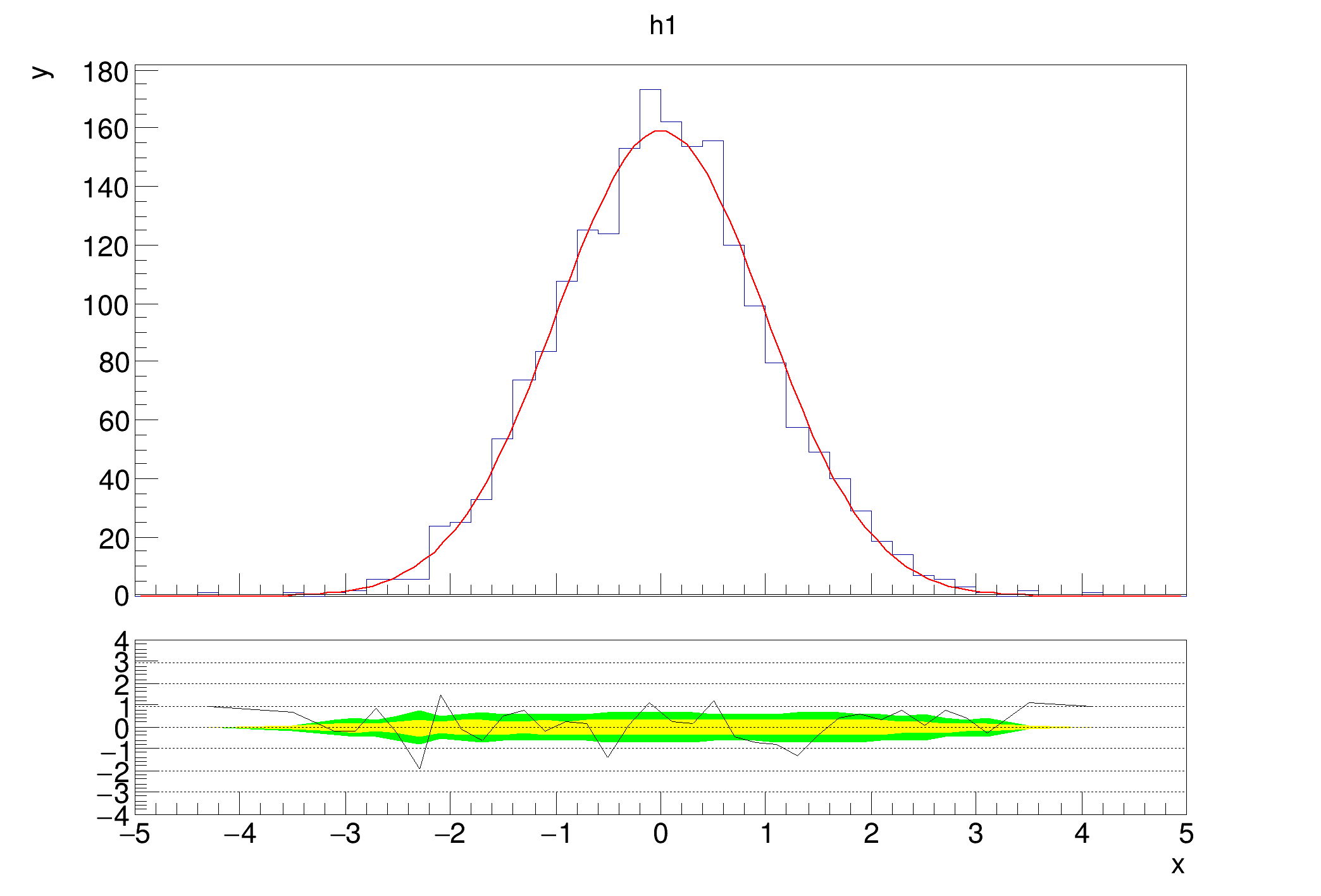Definition at line 1668 of file TRatioPlot.cxx.

## ◆ SetH1DrawOpt()

 void TRatioPlot::SetH1DrawOpt ( Option_t * opt )

Sets the drawing option for h1.

Definition at line 338 of file TRatioPlot.cxx.

## ◆ SetH2DrawOpt()

 void TRatioPlot::SetH2DrawOpt ( Option_t * opt )

Sets the drawing option for h2.

Definition at line 346 of file TRatioPlot.cxx.

## ◆ SetInsetWidth()

 void TRatioPlot::SetInsetWidth ( Double_t width )

Set the inset on the outer sides of all the pads.

It's used to make the outer pad draggable.

Definition at line 1630 of file TRatioPlot.cxx.

## ◆ SetLeftMargin()

 void TRatioPlot::SetLeftMargin ( Float_t margin )

Sets the left margin of both pads.

Parameters
 margin The new margin

Definition at line 481 of file TRatioPlot.cxx.

## ◆ SetLowBottomMargin()

 void TRatioPlot::SetLowBottomMargin ( Float_t margin )

Sets the bottom margin of the lower pad.

Parameters
 margin The new margin

Definition at line 471 of file TRatioPlot.cxx.

## ◆ SetLowTopMargin()

 void TRatioPlot::SetLowTopMargin ( Float_t margin )

Sets the top margin of the lower pad.

Parameters
 margin The new margin

Definition at line 460 of file TRatioPlot.cxx.

protected

Sets the margins of all the pads to the value specified in class members.

This one is called whenever those are changed, e.g. in setters

Definition at line 1374 of file TRatioPlot.cxx.

## ◆ SetRightMargin()

 void TRatioPlot::SetRightMargin ( Float_t margin )

Sets the right margin of both pads.

Parameters
 margin The new margin

Definition at line 492 of file TRatioPlot.cxx.

## ◆ SetSeparationMargin()

 void TRatioPlot::SetSeparationMargin ( Float_t margin )

Sets the margin that separates the two pads.

The margin is split according to the relative sizes of the pads

Parameters
 margin The new margin
void ratioplot6() {
auto c1 = new TCanvas("c1", "fit residual simple");
auto h1 = new TH1D("h1", "h1", 50, -5, 5);
h1->FillRandom("gaus", 5000);
TFitResultPtr fitres = h1->Fit("gaus", "S0");
h1->Sumw2();
h1->GetXaxis()->SetTitle("x");
h1->GetYaxis()->SetTitle("y");
auto rp1 = new TRatioPlot(h1, "errfunc");
rp1->SetGraphDrawOpt("L");
rp1->SetSeparationMargin(0.0);
rp1->Draw();
rp1->GetLowerRefGraph()->SetMinimum(-2);
rp1->GetLowerRefGraph()->SetMaximum(2);
}
Provides an indirection to the TFitResult class and with a semantics identical to a TFitResult pointe...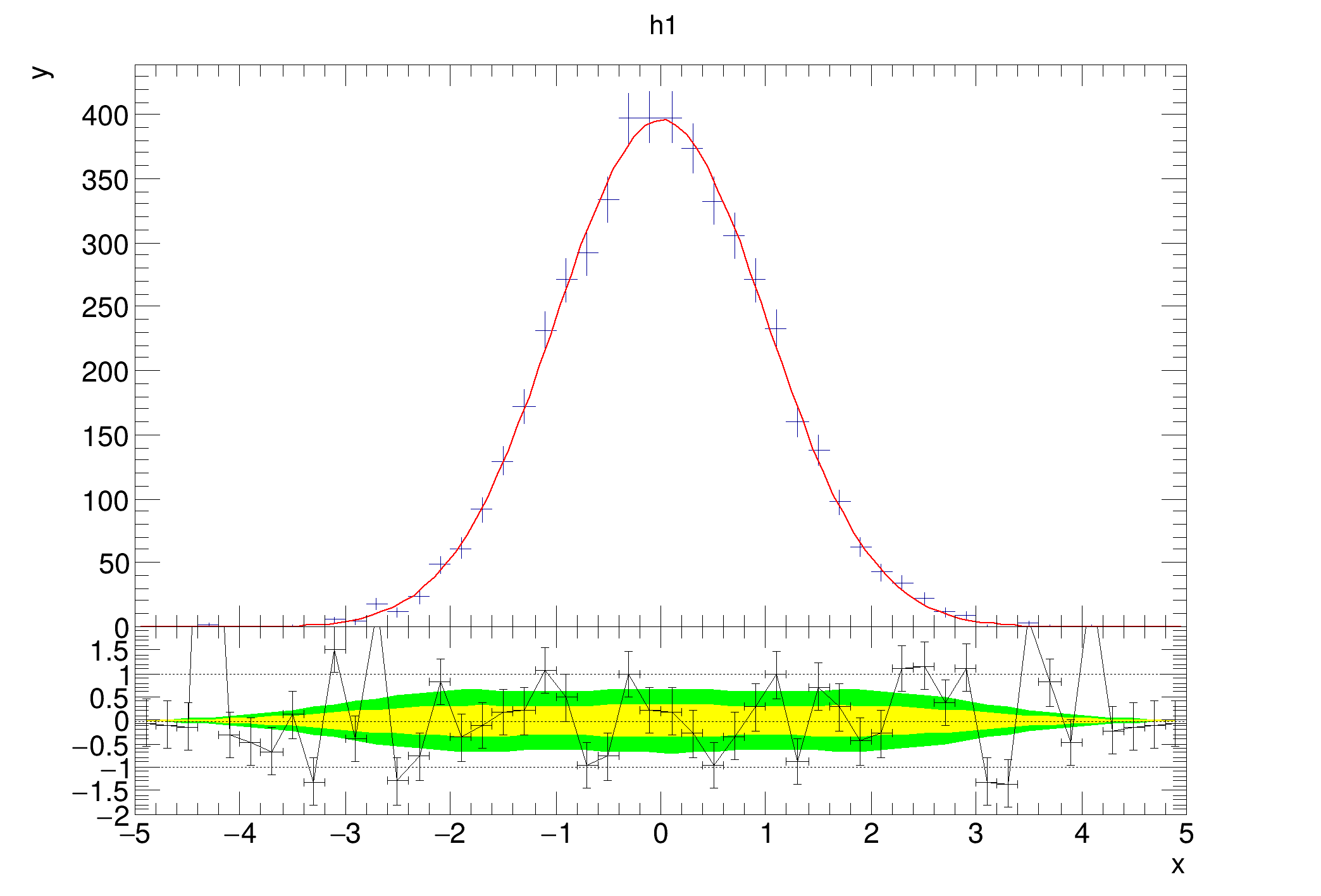Definition at line 508 of file TRatioPlot.cxx.

## ◆ SetSplitFraction()

 void TRatioPlot::SetSplitFraction ( Float_t sf )

Set the fraction of the parent pad, at which the to sub pads should meet.

Definition at line 1609 of file TRatioPlot.cxx.

## ◆ SetUpBottomMargin()

 void TRatioPlot::SetUpBottomMargin ( Float_t margin )

Sets the bottom margin of the upper pad.

Parameters
 margin The new margin

Definition at line 449 of file TRatioPlot.cxx.

protectedvirtual

Definition at line 374 of file TRatioPlot.cxx.

## ◆ SetUpTopMargin()

 void TRatioPlot::SetUpTopMargin ( Float_t margin )

Sets the top margin of the upper pad.

Parameters
 margin The new margin

Definition at line 438 of file TRatioPlot.cxx.

## ◆ Streamer()

 void TRatioPlot::Streamer ( TBuffer & R__b )
overridevirtual

Stream an object of class TObject.

Reimplemented from TObject.

## ◆ StreamerNVirtual()

 void TRatioPlot::StreamerNVirtual ( TBuffer & ClassDef_StreamerNVirtual_b )
inline

Definition at line 264 of file TRatioPlot.h.

Slot that handles common resizing of upper and lower pad.

Definition at line 1562 of file TRatioPlot.cxx.

## ◆ SyncAxesRanges()

 void TRatioPlot::SyncAxesRanges ( )
protectedvirtual

Syncs the axes ranges from the shared ones to the actual ones.

Definition at line 839 of file TRatioPlot.cxx.

protectedvirtual

Figures out which pad margin has deviated from the stored ones, to figure out what the new nominal is and set the other pad to it subsequently.

Definition at line 1391 of file TRatioPlot.cxx.

## ◆ UnZoomed()

 void TRatioPlot::UnZoomed ( )

Slot for the UnZoomed signal that was introduced to TAxis.

Definition at line 1546 of file TRatioPlot.cxx.

## ◆ fC1

 Double_t TRatioPlot::fC1 = 1.
protected

Stores the scale factor for h1 (or THStack sum)

Definition at line 104 of file TRatioPlot.h.

## ◆ fC2

 Double_t TRatioPlot::fC2 = 1.
protected

Stores the scale factor for h2.

Definition at line 105 of file TRatioPlot.h.

## ◆ fCi1Color

 Color_t TRatioPlot::fCi1Color = kYellow
protected

Stores the color for the 1 sigma band.

Definition at line 96 of file TRatioPlot.h.

## ◆ fCi2Color

 Color_t TRatioPlot::fCi2Color = kGreen
protected

Stores the color for the 2 sigma band.

Definition at line 97 of file TRatioPlot.h.

## ◆ fCl1

 Double_t TRatioPlot::fCl1 = 0.6827
protected

Stores the confidence level for the inner confidence interval band.

Definition at line 101 of file TRatioPlot.h.

## ◆ fCl2

 Double_t TRatioPlot::fCl2 = 0.9545
protected

Stores the confidence level for the outer confidence interval band.

Definition at line 102 of file TRatioPlot.h.

## ◆ fConfidenceInterval1

 TGraphErrors* TRatioPlot::fConfidenceInterval1 = nullptr
protected

Stores the graph for the 1 sigma band.

Definition at line 94 of file TRatioPlot.h.

## ◆ fConfidenceInterval2

 TGraphErrors* TRatioPlot::fConfidenceInterval2 = nullptr
protected

Stores the graph for the 2 sigma band.

Definition at line 95 of file TRatioPlot.h.

## ◆ fErrorMode

 Int_t TRatioPlot::fErrorMode = TRatioPlot::ErrorMode::kErrorSymmetric
protected

Stores the error mode, sym, asym or func.

Definition at line 84 of file TRatioPlot.h.

## ◆ fFitDrawOpt

 TString TRatioPlot::fFitDrawOpt = ""
protected

Stores draw option for the fit function in the fit residual case.

Definition at line 89 of file TRatioPlot.h.

## ◆ fFitResult

 TFitResult* TRatioPlot::fFitResult = nullptr
protected

Stores the explicit fit result given in the fit residual case. Can be 0.

Definition at line 107 of file TRatioPlot.h.

## ◆ fGraphDrawOpt

 TString TRatioPlot::fGraphDrawOpt = ""
protected

Stores draw option for the lower plot graph given in constructor.

Definition at line 88 of file TRatioPlot.h.

## ◆ fGridlinePositions

 std::vector TRatioPlot::fGridlinePositions
protected

Stores the y positions for the gridlines.

Definition at line 123 of file TRatioPlot.h.

## ◆ fGridlines

 std::vector TRatioPlot::fGridlines
protected

Keeps TLine objects for the gridlines.

Definition at line 122 of file TRatioPlot.h.

## ◆ fH1

 TH1* TRatioPlot::fH1 = nullptr
protected

Stores the primary histogram.

Definition at line 79 of file TRatioPlot.h.

## ◆ fH1DrawOpt

 TString TRatioPlot::fH1DrawOpt = ""
protected

Stores draw option for h1 given in constructor.

Definition at line 86 of file TRatioPlot.h.

## ◆ fH2

 TH1* TRatioPlot::fH2 = nullptr
protected

Stores the secondary histogram, if there is one.

Definition at line 80 of file TRatioPlot.h.

## ◆ fH2DrawOpt

 TString TRatioPlot::fH2DrawOpt = ""
protected

Stores draw option for h2 given in constructor.

Definition at line 87 of file TRatioPlot.h.

## ◆ fHideLabelMode

 Int_t TRatioPlot::fHideLabelMode = TRatioPlot::HideLabelMode::kHideLow
protected

Stores which label to hide if the margin is to narrow, if at all.

Definition at line 125 of file TRatioPlot.h.

## ◆ fHistDrawProxy

 TObject* TRatioPlot::fHistDrawProxy = nullptr
protected

The object which is actually drawn, this might be TH1 or THStack.

Definition at line 81 of file TRatioPlot.h.

## ◆ fInsetWidth

 Float_t TRatioPlot::fInsetWidth = 0.0025
protected

Definition at line 137 of file TRatioPlot.h.

protected

Keeps track whether pads are updating during resizing.

Definition at line 140 of file TRatioPlot.h.

## ◆ fIsUpdating

 Bool_t TRatioPlot::fIsUpdating = kFALSE
protected

Keeps track of whether its currently updating to reject other calls until done.

Definition at line 139 of file TRatioPlot.h.

## ◆ fLeftMargin

 Float_t TRatioPlot::fLeftMargin = 0.1
protected

Stores the common left margin of both pads.

Definition at line 134 of file TRatioPlot.h.

## ◆ fLowBottomMargin

 Float_t TRatioPlot::fLowBottomMargin = 0.3
protected

Stores the bottom margin of the lower pad.

Definition at line 132 of file TRatioPlot.h.

## ◆ fLowerGXaxis

 TGaxis* TRatioPlot::fLowerGXaxis = nullptr
protected

Lower graphical x axis.

Definition at line 111 of file TRatioPlot.h.

## ◆ fLowerGXaxisMirror

 TGaxis* TRatioPlot::fLowerGXaxisMirror = nullptr
protected

Lower mirror of the x axis.

Definition at line 115 of file TRatioPlot.h.

## ◆ fLowerGYaxis

 TGaxis* TRatioPlot::fLowerGYaxis = nullptr
protected

Lower graphical y axis.

Definition at line 113 of file TRatioPlot.h.

## ◆ fLowerGYaxisMirror

 TGaxis* TRatioPlot::fLowerGYaxisMirror = nullptr
protected

Lower mirror of the y axis.

Definition at line 117 of file TRatioPlot.h.

protected

The pad which contains the calculated lower plot part.

Definition at line 76 of file TRatioPlot.h.

## ◆ fLowTopMargin

 Float_t TRatioPlot::fLowTopMargin = 0.05
protected

Stores the top margin of the lower pad.

Definition at line 131 of file TRatioPlot.h.

## ◆ fLowYaxis

 TAxis* TRatioPlot::fLowYaxis = nullptr
protected

Clone of the lower y axis.

Definition at line 120 of file TRatioPlot.h.

## ◆ fMode

 Int_t TRatioPlot::fMode = 0
protected

Stores which calculation is supposed to be performed as specified by user option.

Definition at line 83 of file TRatioPlot.h.

## ◆ fOption

 TString TRatioPlot::fOption = ""
protected

Stores the option which is given in the constructor as a string.

Definition at line 85 of file TRatioPlot.h.

protected

Stores the pad the ratio plot was created in.

Definition at line 74 of file TRatioPlot.h.

## ◆ fRatioGraph

 TGraph* TRatioPlot::fRatioGraph = nullptr
protected

Stores the lower plot's graph.

Definition at line 93 of file TRatioPlot.h.

## ◆ fRightMargin

 Float_t TRatioPlot::fRightMargin = 0.1
protected

Stores the common right margin of both pads.

Definition at line 135 of file TRatioPlot.h.

## ◆ fSharedXAxis

 TAxis* TRatioPlot::fSharedXAxis = nullptr
protected

X axis that stores the range for both plots.

Definition at line 109 of file TRatioPlot.h.

## ◆ fShowConfidenceIntervals

 Bool_t TRatioPlot::fShowConfidenceIntervals = kTRUE
protected

Stores whether to show the confidence interval bands. From Draw option.

Definition at line 99 of file TRatioPlot.h.

## ◆ fShowGridlines

 Bool_t TRatioPlot::fShowGridlines = kTRUE
protected

Stores whether to show the gridlines at all.

Definition at line 124 of file TRatioPlot.h.

## ◆ fSplitFraction

 Float_t TRatioPlot::fSplitFraction = 0.3
protected

Stores the fraction at which the upper and lower pads meet.

Definition at line 91 of file TRatioPlot.h.

protected

The Pad that drawn on top on the others to have consistent coordinates.

Definition at line 77 of file TRatioPlot.h.

## ◆ fUpBottomMargin

 Float_t TRatioPlot::fUpBottomMargin = 0.05
protected

Stores the bottom margin of the upper pad.

Definition at line 130 of file TRatioPlot.h.

## ◆ fUpperGXaxis

 TGaxis* TRatioPlot::fUpperGXaxis = nullptr
protected

Upper graphical x axis.

Definition at line 110 of file TRatioPlot.h.

## ◆ fUpperGXaxisMirror

 TGaxis* TRatioPlot::fUpperGXaxisMirror = nullptr
protected

Upper mirror of the x axis.

Definition at line 114 of file TRatioPlot.h.

## ◆ fUpperGYaxis

 TGaxis* TRatioPlot::fUpperGYaxis = nullptr
protected

Upper graphical y axis.

Definition at line 112 of file TRatioPlot.h.

## ◆ fUpperGYaxisMirror

 TGaxis* TRatioPlot::fUpperGYaxisMirror = nullptr
protected

Upper mirror of the y axis.

Definition at line 116 of file TRatioPlot.h.

protected

The pad which contains the upper plot part.

Definition at line 75 of file TRatioPlot.h.

## ◆ fUpTopMargin

 Float_t TRatioPlot::fUpTopMargin = 0.1
protected

Stores the top margin of the upper pad.

Definition at line 129 of file TRatioPlot.h.

## ◆ fUpYaxis

 TAxis* TRatioPlot::fUpYaxis = nullptr
protected

Clone of the upper y axis.

Definition at line 119 of file TRatioPlot.h.

Libraries for TRatioPlot:[legend]

The documentation for this class was generated from the following files: